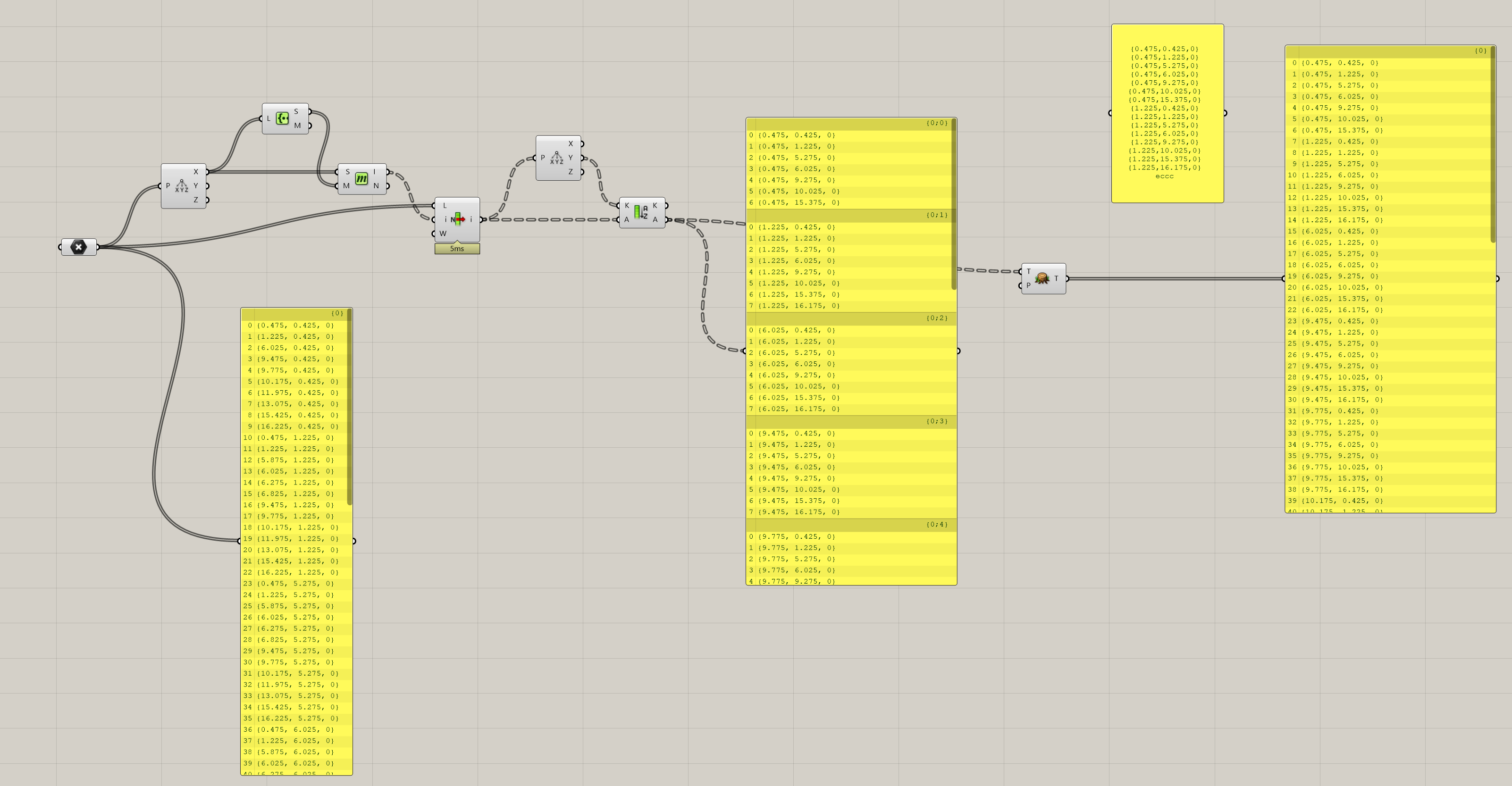# Sorted points list by X coordinate and then by Y coordinate

Hi, I have a points list and I want to sort it by X coordinate first and then by Y coordinate.
If I use the “sort list” component I only able to sort it according to X coordinate or to Y coordinate.
Someone can help me?
Thanks.

Test.gh (27.7 KB)

VoilàTest.gh (26.8 KB)

1 Like

@martinsiegrist Thank you… Do you also know how to do it with gh python??

Sorry, no.

You can also do this with the Sort Points component.

This shows how to do the same thing with a GhPython or C# script.
sort_points.gh (15.4 KB)

-Kevin

1 Like

Sorting with @kev.r solutions seem to fail on the points provided further above.

Using my Grasshopper sorting above and string formatting, the coordinates look like this:

Looks like the values provided should be rounded before sorting:

Test.gh (30.1 KB)

1 Like

I usually do something like this in Python:
(Edit* I made a small change)
Test_v3.gh (8.4 KB)

1 Like

To use Sort Points, you could also round in Python.

``````import rhinoscriptsyntax as rs
rnd = 3

npnts = [rs.AddPoint(round(i.X,rnd),round(i.Y,rnd),round(i.Z,rnd)) for i in pnts]
npnts = rs.coerce3dpointlist(npnts)

a = rs.SortPoints(npnts, True,0)
``````
1 Like

I usually use this pattern when sorting by an object property:

Yes, old trick. You can round point coordinates to any precision using an expression: `round(Pt,P)`

sort_points_2022Nov1a.gh (5.7 KB)

Thanks

Translate this (in C#): pList = pList.OrderBy(x=>x.X).ThenBy(x=>x.Y).ToList();

ot for rounded coords use: (x=>Math.Round(x.X, decimals)) , (x=>Math.Round(x.Y, decimals))

1 Like

By the way, you can change the way points are sorted like this (Y, X, Z):

sort_points_2022Nov1b.gh (8.7 KB)

Or sort by Z first, then Y, then X.

1 Like